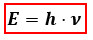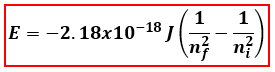Chemistry Practice Problems Bohr and Balmer Equations Practice Problems Solution: Calculate the frequency of the light emitted by a ...

🤓 Based on our data, we think this question is relevant for Professor Sandusky's class at URI.

# Solution: Calculate the frequency of the light emitted by a hydrogen atom during a transition of its electron from the n = 3 to n = 1 energy level, based on the Bohr theory. Use the equation En = -2.18 x 10 -18 J [(1/nf2)-(1/ni2)]a. 2.92 x 1015 s-1b. 3.56 x 1014 s-1c. 2.92 x 1014 s-1d. 1.17 x 1015 s-1

###### Problem

Calculate the frequency of the light emitted by a hydrogen atom during a transition of its electron from the n = 3 to n = 1 energy level, based on the Bohr theory. Use the equation En = -2.18 x 10 -18 J [(1/nf2)-(1/ni2)]

a. 2.92 x 1015 s-1

b. 3.56 x 1014 s-1

c. 2.92 x 1014 s-1

d. 1.17 x 1015 s-1

###### Solution

We will calculate the frequency of the light emitted by a hydrogen atom from the energy using the following equation:E = energy, J/photon
h = Planck' s constant = 6.626x10-34 J
s
ν = frequency

Let’s first calculate the energy of the hydrogen atom using the given Bohr Equation:ni = initial energy level
nf = final energy levelView Complete Written Solution

Bohr and Balmer Equations

Bohr and Balmer Equations

#### Q. What is the wavelength of the light emitted from a hydrogen atom when an electron moves from the n = 6 to n = 2 energy level?R = 1.096776 x 107 m-1.A....

Solved • Mon May 29 2017 08:41:26 GMT-0400 (EDT)

Bohr and Balmer Equations

#### Q. An excited hydrogen atom emits a photon with a frequency of 1.141 x 10 14 Hz to reach the n = 4 state. From what state did the electron originate?  a...

Solved • Thu May 25 2017 17:59:48 GMT-0400 (EDT)

Bohr and Balmer Equations

#### Q. Suppose energy is delivered to atoms of fluorine, chlorine, bromine, and iodine sufficient to cause each atom’s outermost electron to jump to the n=7 ...

Solved • Mon Oct 24 2016 12:40:40 GMT-0400 (EDT)

Bohr and Balmer Equations

#### Q. An electron in a hydrogen atom moves from the n = 2 to n = 5 level. What is the wavelength of the photon that corresponds to this transition and is th...

Solved • Tue Oct 18 2016 14:30:41 GMT-0400 (EDT)